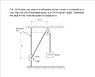# Statics questions

hjkklm
The units are different.
One is the force unit, and one is the length unit.
Then how to find the h?

Question is attached in the file

thanks

#### Attachments

•Mechanics Q_1.jpeg
25.3 KB · Views: 462

Homework Helper

hjkklm
I intend to find the all forces and then find the length through the force quantity.
I will find the forces component firstly. x-component=150cosθ N (h cm), y-component=150sinθ N (40cm)
150cosθ N= 170N <----but it is math error or no solution

Then I can't find, help please.